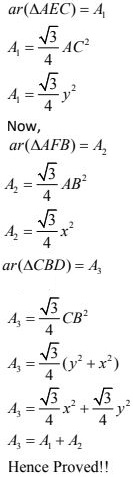# Chapter 6 Triangles NCERT Exemplar Solutions Exercise 6.4 Class 10 MathsChapter Name NCERT Maths Exemplar Solutions for Chapter 6 Triangles Exercise 6.4 Book Name NCERT Exemplar for Class 10 Maths Other Exercises Exercise 6.1Exercise 6.2Exercise 6.3 Related Study NCERT Solutions for Class 10 Maths

### Exercise 6.4 Solutions

1. In Fig., if ∠A = ∠C, AB = 6 cm, BP = 15 cm, AP = 12 cm and CP = 4 cm, then find the lengths of PD and CD.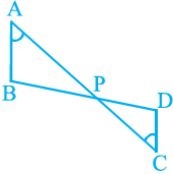Solution

We have,
∠A = ∠C,
AB = 6 cm,
BP = 15 cm,
AP = 12 cm and
CP = 4 cm
In ∆APB and ∆CPD,
∠A = ∠C  [given]
∠APB = ∠CPD  [vertically opposite angles]
∆APB ~ ∆CPD  [by AA similarity criterion]
AP/CP = PB/PD = AB/CD
⇒ 12/4 = 15/PD = 6/CD
So,
12/4 = 15/PD
⇒ PD = 5 cm
Also,
12/4 = 6/CD
⇒ CD = 2 cm
Therefore, length of PD is 5 cm and length of CD is 2 cm.

2. It is given that ∆ ABC ~ ∆ EDF such that AB = 5 cm, AC = 7 cm, DF= 15 cm and DE = 12 cm. Find the lengths of the remaining sides of the triangles.

Solution

We have,
∆ABC ~ ∆EDF, so the corresponding sides of ∆ABC and ∆EDF are in the same ratio
AB/ED = AC/EF = BC/DF  ...(i)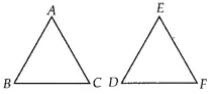Also, we have,
AB = 5 cm,
AC = 7 cm,
DF= 15 cm and
DE = 12 cm
Putting value in AB/ED = AC/EF = BC/DF ,
5/12 = 7/EF = BC/15
So,
5/12 = 7/EF
⇒ EF = 16.8 cm
Also,
5/12 = BC/15
⇒ BC = 6.25 cm
So, lengths of the remaining sides of the triangles are EF =  16.8 cm and
BC = 6.25 cm.

3. Prove that if a line is drawn parallel to one side of a triangle to intersect the other two sides, then the two sides are divided in the same ratio.

Solution

Let us take ∆ABC in which a line DE parallel to BC intersects AB at D and AC at E.
To prove: DE divides the two sides in the same ratio.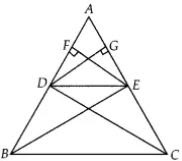Construction :
Join BE and CD
EF ⊥ AB
DG ⊥ AC
Proof :
Also,
ar(ADE)/ar(DEC) = (1/2 × AE × GD)/(1/2 × EC × GD)
= AE/EC ...(ii)
As,
∆BDE and ∆DEC lie between the same parallel lines DE and BC and on the same base DE
So,
ar(∆BDE) = ar(∆DEC)  ...(iii)
From Eqs. (i), (ii) and (iii),
Hence proved

4. In Fig., if PQRS is a parallelogram and AB|| PS, then prove that OC || SR.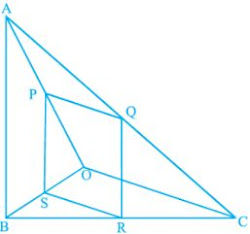Solution

We have,
PQRS is a parallelogram, so PQ || SR and PS || QR.
Also,
AB || PS.
To prove :
OC || SR
Proof : In ΔOPS and ΔOAB, PS || AB
∠POS = ∠AOB  [common angle]
∠OSP = ∠OBA  [corresponding angles]
∆OPS ~ ∆OAB   [by AA similarity criterion]
Then PS/AB = OS/OB  ...(i)
In ∆CQE and ∆CAB, QR || PS || AB
∠QCR = ∠ACB  [common angle]
∠CRQ = ∠CBA   [corresponding angles]
So,
∆CQR ~ ∆CAB
QR/AB = CR/OB
PS/AB = CR/OB  ...(ii)
(PS = QR, opposite sides of parallelogram)
From (i) and (ii)
OS/OB = CR/CB
or,
OB/OS = CB/CR
Subtracting 1 from both sides, we get,
(OB/OS) - 1 = (CB/CR) - 1
⇒ (OB - OS)/OS = (CB - CR)/CR
⇒ BS/OS = BR/CR
By using converse of basic proportionality theorem, SR || OC.
Hence, proved

5. A 5 m long ladder is placed leaning towards a vertical wall such that it reaches the wall at a point 4 m high. If the foot of the ladder is moved 1.6 m towards the wall, then find the distance by which the top of the ladder would slide upwards on the wall.

Solution

Taking AC be the ladder of length 5 m and BC = 4 m be the height of the wall, which ladder is placed.
If the foot of the ladder is moved 1.6 m towards the wall so, AD = 1.6 m, then the ladder is slide upward i.e., CE = x m.
In right angled ∆ABC,
AC2 = AB2 + BC2  [Using Pythagoras theorem]
⇒ (5)2 = (AB)2 + (4)2
⇒ AB2 = 25 - 16 = 9
⇒ AB = 3m
Now,
= 3 - 1.6
= 1.4 m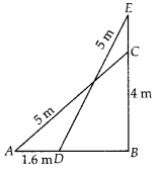In right angled ∆EBD,
ED2 = EB2 + BD2
[using Pythagoras theorem]
(5)2 = (EB)2 + (1.4)2
[ ∵ BD = 1.4 m]
25 = (EB)2 + 1.96
⇒ (EB)2 = 25 – 1.96 = 23.04
⇒ EB = 4.8
Now,
EC = EB – BC
= 4.8 – 4
= 0.8
Therefore, the top of the ladder would slide upwards on the wall at distance is 0.8 m.

6. For going to a city B from city A, there is a route via city C such that AC⊥CB, AC = 2 x km and CB = 2 (x + 7) km. It is proposed to construct a 26 km highway which directly connects the two cities A and B. Find how much distance will be saved in reaching city B from city A after the construction of the highway.

Solution

We have,
AC ⊥ CB,
AC = 2 × km,
CB = 2(x + 7) km and AB = 26 km
On drawing the figure, we get the right angle ∆ACB right angle at C.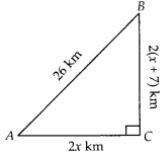Now,
In ∆ACB, by Pythagoras theorem,
AB2 = AC2 + BC2
⇒ (26)2 = (2x)2 + {2(x + 7)}2
⇒ 676 = 4x2 + 4(x2 + 49 + 11x)
⇒ 676 = 4x2 + 4x2 + 196 + 56x
⇒ 676 = 8x2 + 56x + 196
⇒ 8x2 + 56x – 480 = 0
On dividing by 8, we get,
x2 + 7x – 60 = 0
⇒ x2 + 12x-5x-60 = 0
⇒ x(x + 12) – 5(x + 12) = 0
⇒ (x + 12)(x – 5) = 0
⇒ x = -12 or, x = 5
As, distance cannot be negative.
x = 5  [∵ x ≠ 12]
Now,
AC = 2x
= 10 km and
BC = 2(x + 7)
= 2(5 + 7)
= 24 km
The distance covered to reach city B from city A via city C = AC + BC = 10 + 24 = 34 km
Distance covered to reach city B from city A after the construction of the highway is
BA = 26 km
So, the required saved distance is 34 – 26= 8 km.

7. A flag pole 18 m high casts a shadow 9.6 m long. Find the distance of the top of the pole from the far end of the shadow.

Solution

Let BC = 18 m be the flag pole and its shadow be AB = 9.6 m. The distance of the top of the pole, C from the far end which is A of the shadow is AC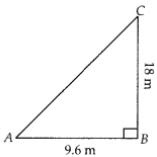In right angled ∆ABC
AC2 = AB2 + BC2  [using Pythagoras theorem]
⇒ AC2 = (9.6)2 + (18)2
⇒ AC2 = 92.16 + 324
⇒ AC2 = 416.16
⇒ AC = 20.4 m
So, the required distance is 20.4 m.

8. A street light bulb is fixed on a pole 6 m above the level of the street. If a woman of height 1.5 m casts a shadow of 3m, find how far she is away from the base of the pole.

Solution

Taking A be the position of the street bulb fixed on a pole AB = 6 m and CD = 1.5 m be the height of a woman and her shadow be ED = 3 m. And distance between pole and woman be x m.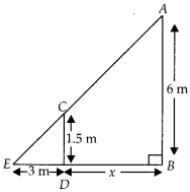In this question, woman and pole both are standing vertically
So,
CD || AB
In ∆CDE and ∆ABE,
∠E = ∠E  [common angle]
∠ABE = ∠CDE  [each equal to 90°]
∆CDE ~ ∆ABE  [by AA similarity criterion]
Then,
ED/EB = CD/AB
⇒ 3/(3 +x) = 1.5/6
⇒ 3×6 = 1.5(3 + x)
⇒ 18 = 1.5 × 3 + 1.5x
⇒ 1.5x = 18 – 4.5
⇒ x = 9 m
So, she is at the distance of 9 m from the base of the pole.

9. In Fig., ABC is a triangle right angled at B and BD ⊥ AC. If AD = 4 cm, and CD = 5 cm, find BD and AB.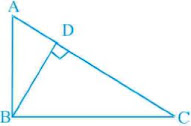Solution

Given,
∆ABC in which ∠B = 90° and
BD ⊥ AC
Also, AD = 4 cm and
CD = 5 cm
In ∆DBA and ∆DCB,
∠ADB = ∠CDB   [each equal to 90°]
and
∠BAD = ∠DBC  [each equal to 90° – ∠C]
∆DBA ~ ∆DCB  [by AA similarity criterion]
So,
DB/DA = DC/DB
⇒ DB2 = DA × DC = 4 × 5
⇒ DB = 2√5 cm
In ΔBDC,
BC2 = BD2 + CD2  (Using Pythagoras theorem)
= (2√5)2  + 52
= 3√5
Also,
ΔDBA ~  ΔDBC
DB/DC = BA/BC
⇒ (2√5)/5 = BA/3√5
⇒ AB = 6 cm

10. 10. In Fig., PQR is a right triangle right angled at Q and QS ⊥ PR . If PQ = 6 cm and PS = 4 cm, find QS, RS and QR.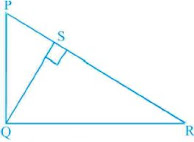Solution

We have,
In ∆PQR,
∠Q = 90°,
QS ⊥ PR and
PQ = 6 cm,
PS = 4 cm
In ∆SQP and ∆SRQ,
∠PSQ = ∠RSQ   [each equal to 90°]
∠SPQ = ∠SQR   [each equal to 90° – ∠R]
∆SQP ~ ∆SRQ   [By AA similarity criterion]
Then, SQ/PS = SR/SQ
SQ2 = PS × SR
In right angled  ΔPSQ,
PQ2 = PS2 + QS2   [using Pythagoras theorem]
⇒ (6)2 = (4)2 + QS2
⇒ 36 = 16 + QS2
⇒ QS2 = 36 – 16 = 20
⇒ QS = 2√5 cm
From eq. (i),
Putting value of PS and QS we get,
RS = 5cm
Now, In QSR,
QR2 = QS2 +SR2
So, putting value of QS and SR we get,
QR = 3√5cm

11. In ∆PQR, PD ⊥ QR such that D lies on QR. If PQ = a, PR = b, QD = c and DR = d, prove that (a + b)(a - b) = (c + d)(c - d).

Solution

Given:
In ∆PQR, PD ⊥ QR,
PQ = a,
PR = b,
QD = c and
DR = d
To prove: (a + b)(a -b) = (c + d)(c – d)
Proof:
In right angled ∆PDQ,
PQ2 = PD2 + QD2  [using Pythagoras theorem]
a2  =  PD2 + c2
PD2 = a2 - c2  ...(i)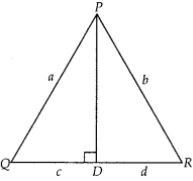In right angled ∆PDR,
PR2 = PD2 + DR2  [Using Pythagoras theorem]
b2 = PD2 + d2
PD2 = b2 - d2  ...(ii)
From Eq. (i) and (ii)
a2 - c2 = b2 - d2
⇒ a2  - b2 = c2 - d2
⇒ (a - b)(a + b) = (c - d)(c + d)
Hence proved.

12. In a quadrilateral ABCD, ∠A + ∠D = 90° . Prove that AC2 + BD2 = AD2 + BC2
[Hint : Produce AB and DC to meet at E.]

Solution
Given :
∠A + ∠D = 90°
To prove : AC2 + BD2 = AD2 + BC2
Construct : Produce AB and CD to meet at E
Also join AC and BD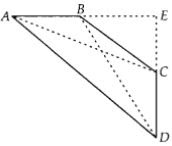Proof:
In ∆AED,
∠A + ∠D = 90°  [given]
∠E = 180° – (∠A + ∠D)
= 90°   [sum of angles of a triangle = 180°]
So, by Pythagoras theorem,
In ∆BEC, by Pythagoras theorem,
BC2 = BE2 + EC2
AD2 + BC2 = AE2 + DE2 + BE2 + CE2  ...(i)
In ∆AEC, by Pythagoras theorem,
AC2 = AE2 + CE2
In ∆BED, by Pythagoras theorem,
BD2 = BE2 + DE2
AC2 + BD2 = AE2 + CE2 + BE2 + DE2  ...(ii)
From Eqs. (i) and (ii)
AC2 + BD2 = AD2 + BC2
Hence proved.

13. In Fig., l  || m and line segments AB, CD and EF are concurrent at point P.
Prove that :  AE/BF = AC/BD = CE/FDSolution

We have, l || m and line segment AB, CD and EF are concurrent at point P
To Prove,
AE/BF = AC/BD = CE/FD
In ΔAPC and ΔBPD,
APC = BPD  (vertically opposite angles)
PAC =PBD  (Alternate angles)
So,
ΔAPC:ΔBPD  (By AA Similarity)
AP/PB = AC/BD = PC/PD
Now,
In ΔAPE and ΔBPF,
APE =BPF   (vertically opposite angles)
PAE =PBF   (Alternate angles)
so,
ΔAPE:ΔBPF  (By AA Similarity)
Now,
In ΔPEC and ΔPFD,
APC = BPD  (vertically opposite angles)
PAC = PBD  (Alternate angles)
so,
ΔPEC:ΔPDF  (By AA Similarity)
PC/PD = PE/PF = EC/FD
So, from above equations,
AP/PB = AC/BD = PE/PF = EC/FD = AE/BF
AE/BF = AC/BD = EC/FD
Hence, proved.

14. In Fig., PA, QB, RC and SD are all perpendiculars to a line l, AB = 6 cm, BC = 9 cm, CD = 12 cm and SP = 36 cm. Find PQ, QR and RS.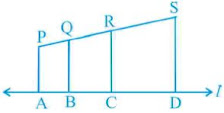Solution

We have,
AB = 6 cm,
BC = 9 cm,
CD = 12 cm and
SP = 36 cm
Also, PA, QB, RC and SD are all perpendiculars to line l,
PA || QB || RC || SD
Using Basic proportionality theorem,
PQ : QR : RS = AB : BC : CD
= 6 : 9 : 12
Taking,
PQ = 6x,
QR = 9x and
RS = 12x
As,
Length of PS = 36 cm
PQ + QR + RS = 36
⇒ 6x + 9x + 12x = 36
⇒ 27x = 36
⇒ x = 4/3
Now,
PQ = 6x
= 6 × 4/3
= 8 cm
QR = 9x
= 9 × 4/3
= 12 cm
RS = 12x
= 12 × 4/3
= 16 cm

15. O is the point of intersection of the diagonals AC and BD of a trapezium ABCD with AB || DC. Through O, a line segment PQ is drawn parallel to AB meeting AD in P and BC in Q. Prove that PO = QO.

Solution

Given ABCD is a trapezium. Diagonals AC and BD are intersect at O.
PQ || AB || DC
To prove : PO = QO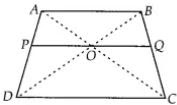Proof:
In ∆ABD and ∆POD,
PO || AB  [as, PQ || AB]
∠D = ∠D  [common angle]
∠ABD = ∠POD  [corresponding angles]
∆ABD ~ ∆POD   [by AA similarity criterion]
Then,
In ∆ABC and ∆OQC,
OQ || AB
∠C = ∠C  [common angle]
∠B AC = ∠QOC  [corresponding angles]
∴ ∆ABC ~ ∆OQC  [by AA similarity criterion]
OQ/AB = QC/BC
OP || DC
AP/PD = OA/OC
In ΔABC,OQ || AB
BQ/QC = OA/OC
Therefore,
AP/PD = BQ/QC
(AP/PD) + 1 = (BQ/QC) + 1
(AP+ PD)/PD = (BQ + QC)/QC
Or,
Also,
OP/AB = QC/BC and OP/AB = OQ/AB
Therefore,
OP = OQ

16. In Fig., line segment DF intersect the side AC of a triangle ABC at the point E such that E is the mid-point of CA and ∠AEF = ∠AFE. Prove that BD/CD = BF/CE
[Hint : Take point G on AB such that CG || DF.]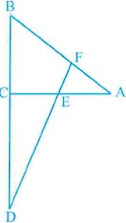Solution
Given ∆ABC, E is the mid-point of CA and ∠AEF = ∠AFE
To prove:
BD/CD = BF/CE
Construction: Take a point G on AB such that CG || DF
Proof: As, E is the mid-point of CA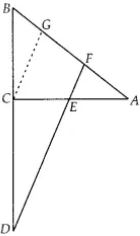CE = AE  ...(i)
In ∆ACG, CG || EF and E is mid-point of CA
So, CE = GF ...(ii) [by mid-point theorem]
Now, in ∆BCG and ∆BDF, CG || DF
BC/CD = BG/GF
⇒ BC/CD = (BF - GF)/GF
⇒ BC/CD = (BF/GF) - 1
⇒ (BC/CD) + 1 = BF/CE  [from (ii)]
⇒ (BC + CD)/CD = BF/CE
⇒ BD/CD = BF/CE

17. Prove that the area of the semicircle drawn on the hypotenuse of a right angled triangle is equal to the sum of the areas of the semicircles drawn on the other two sides of the triangle.
Solution
ABC is a right triangle, right angled at B in which
AB = y,
BC = x
We will draw three semi-circles are drawn on the sides AB,BC and AC, respectively with
diameters AB,BC and AC, respectively.
Again,
Taking area of circles with diameters AB, BC and AC are respectively A1, A2 and A3
To prove : A3 = A1 + A2
Proof :
In ∆ABC, by Pythagoras theorem,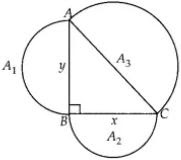AC2 = AB2 + BC2
⇒ AC2 = y2 + x2
⇒ AC = √(y2 + x2 )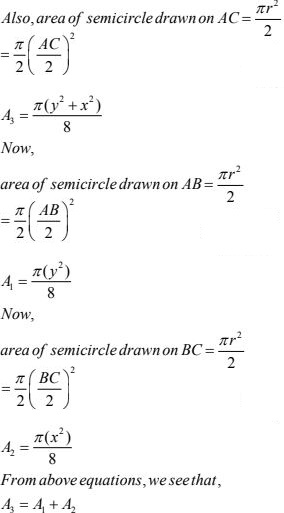Hence Proved.

18. Prove that the area of the equilateral triangle drawn on the hypotenuse of a right angled triangle is equal to the sum of the areas of the equilateral triangles drawn on the other two sides of the triangle..
Solution
BAC is a right triangle in which ∠A is right angle and
AC = y,
AB = x
Now we draw three equilateral triangles on the three sides of ∆ABC,
∆AEC,
∆AFB and
∆CBD
Let us assume area of triangles made on AC, AB and BC are A1 , A2  and A3  respectively.
We need to prove that,
A3 = A1 + A2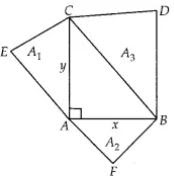Proof :
In ∆CAB, using Pythagoras theorem,
BC2 = AC2 + AB2
BC2 = y2 + x2
BC = √(y2 + x2 )
Also Area of equilateral triangle = (√3/4)a2
Now we calculate the area A1 , A2 and A3 respectively